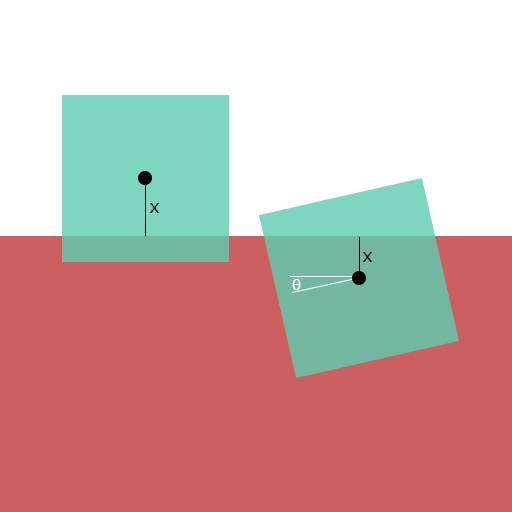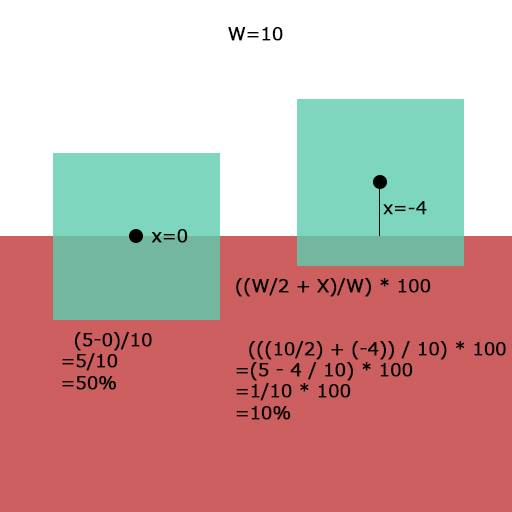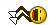# Finding percentage of volume/area in a cube/square

Okay, so this is quite hard to explain in words so I will use pictures.Suppose you have a Square with the length of W
You also know the distance from the pink surface to the center of the square is X. The rotation of the square is theta. As shown in the picture above...

So the problem is: Is it possible to find how much of the pink is inside the square using X and theta using percentage? For example I know that if the rotation is 0, and the distance from the pink to the center of the cube is 0, it will be 50%(shown in the bottom picture)... However, when the angle changes, I do not know how to solve it....So is this even possible? I have a feeling it is but its very complicated
ALSO, if it is possible, can you do this for a cube?

Thanks

## Answers and Replies

mfb
Mentor
You can draw it, therefore it is possible to calculate it.

If the square is partially inside like in the upper right case, it is relatively easy to find the answer - draw a line through the "upper end" of your line "x" that is parallel to the top of the square. It will create two triangles of the same size - one inside, one outside the red area. You can imagine to exchange them, then your problem is reduced to the lower right case, you just have to find the distance between the center and this new line. This can be done with trigonometry.

If two adjacent sides of your square are partially (or outside) in the red area, it gets more complicated, but again trigonometry helps.

A cube can have two different tilt angles and more cases, in the general case that gets messy.

You can draw it, therefore it is possible to calculate it.

That seems like a very profound fact, do you have any supporting evidence? Is there truly an intrinsic connection between drawing something and calculating it?

I have done a project similar to this - It's definitely possible to find the area percentage analytically, and even if it wasn't you could also find it numerically. Like mfb said, you use trigonometry to do it.

mfb
Mentor
That seems like a very profound fact, do you have any supporting evidence? Is there truly an intrinsic connection between drawing something and calculating it?
Well, a numeric solution is obvious - you can simply count pixels.
For analytic solutions with straight lines only (!): if you know the coordinates of all lines, you can calculate the intersections and get analytic values for them. You can then divide all areas into triangles and calculate the area of each one.
In general, this is not possible if you have some curves, but then you can still get a numeric approximation.

•zoki85
Well, it depends on the kind of curve, mfb: The problem of a circle overlap percentage is even easier! (Just take the area of the circular segment)

mfb
Mentor
In general, this is not possible if you have some curves, but then you can still get a numeric approximation.
Sure, there are many cases where it is possible.

Well, a numeric solution is obvious - you can simply count pixels.